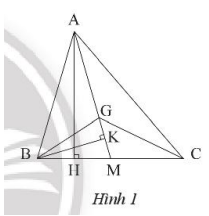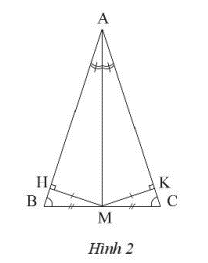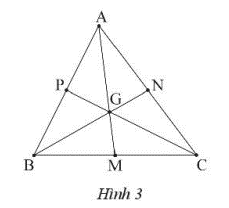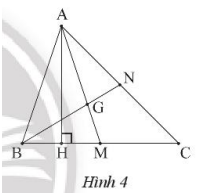## Solving SBT Lesson 7: Properties of the three medians of a triangle (C8 SBT Math 7 Horizons) – Math Book

SBT Solution Lesson 7: Properties of the three medians of a triangle (C8 SBT Math 7 Horizon)
===========

### Solution 1 page 60 SBT Math 7 Creative horizon episode 2 – CTST

Let ABC be a triangle with median AM and G as the centroid. Prove:

a) $${S_{AMB}} = {S_{AMC}}$$

b) $${S_{ABG}} = 2{S_{BMG}}$$

c) $${S_{GAB}} = {S_{GBC}} = {S_{GAC}}$$

Detailed instructions for solving Lesson 1

Solution method

Compare the altitudes and corresponding bases of the triangles

Detailed explanationa) Draw altitude AH of triangle ABC.

Two triangles AMB and AMC have the same altitude AH and the same base side: BM = CM

Derive: $${S_{AMB}} = {S_{AMC}}$$(because $${S_{AMB}} = \frac{1}{2}.AH.BM{;^{}}{) S_{AMC}} = \frac{1}{2}.AN.CM$$)

b) Draw altitude BK of triangle BGM.

Two triangles ABG and BMG have the same altitude BK and base side AG = 2MG.

Derive: $${S_{ABG}} = \frac{1}{2}.BK.AG = \frac{1}{2}.BK.2MG = 2.\frac{1}{2}.BK .MG = 2{S_{BMG}}$$

c) We have:

$${S_{ABG}} = \frac{2}{3}{S_{ABM}} = \frac{1}{3}{S_{ABC}}$$

Similarly: $${S_{ACG}} = \frac{2}{3}{S_{ACM}} = \frac{1}{3}{S_{ABC}}$$

Derive: $${S_{BCG}} = \frac{1}{3}{S_{ABC}}$$

So: $${S_{GAB}} = {S_{GBC}} = {S_{GAC}} = \frac{1}{3}{S_{ABC}}$$

–>

— *****

### Solution 2 page 60 SBT Math 7 Creative horizon episode 2 – CTST

Let ABC be a triangle with median AM and bisector of angle A. Prove that triangle ABC is an isosceles triangle.

Detailed instructions for solving Lesson 2

Solution method

– Prove: $$\Delta AMH = \Delta AMK$$ infer: MH = MK

– Prove: $$\widehat B = \widehat C$$ deduce triangle ABC is isosceles

Detailed explanationDraw altitude MH of triangle AMB and draw altitude MK of triangle AMC.

We have $$\Delta AMH = \Delta AMK$$ (because two right triangles have a common hypotenuse AM, and an acute angle is equal)

Infer: MH = MK.

From that, we have: $$\Delta MBH = \Delta MCK$$ (two right-angled triangles sharing hypotenuse Am and one equal right angle side: MH = MK)

Derive $$\widehat B = \widehat C$$

So triangle ABC is an isosceles triangle at A.

–>

— *****

### Solution 3 page 60 SBT Math 7 Creative horizon episode 2 – CTST

Let ABC be a triangle with two medians AM and CN intersecting at G.

a) Knowing AM = 12 cm, calculate AG.

b) Knowing GN = 3 cm, calculate CN.

c) Find x knowing AG = 3x – 4, GM = x.

Detailed instructions for solving Lesson 3

Solution method

Using the property of three medians of a triangle

Detailed explanation

a) AM = 12 cm, so: $$AG = \frac{2}{3}AM = \frac{2}{3}.12 = 8cm$$

b) GN = 3cm inferred: CN = 3GN = 3.3= 9cm

c) AG = 2GM, infer: 3x – 4 = 2x infer x = 4

–>

— *****

### Solution 4 page 60 SBT Math 7 Creative horizon episode 2 – CTST

Let ABC be a triangle with three medians AM, BN, CP concurrent at G. Prove: $$GA + GB + GC = \frac{2}{3}\left( {AM + BN + CP} \right)$$

Detailed instructions for solving Lesson 4

Solution method

Using property of three medians

Detailed explanationWe have G as the centroid of triangle ANC, so we have:

$$GA = \frac{2}{3}AM;GB = \frac{2}{3}BN;GC = \frac{2}{3}CP$$

Derive: $$GA + GB + GC = \frac{2}{3}\left( {AM + BN + CP} \right)$$

–>

— *****

### Solve problems 5 pages 60 SBT Math 7 Creative horizon episode 2 – CTST

Let ABC be a triangle with two medians AM and BN intersecting at G. Draw AH perpendicular to BC at H. Show HB = HM. Prove:

a) $$\Delta ABH = \Delta AMH$$

b) $$AG = \frac{2}{3}AB$$

Detailed instructions for solving Lesson 5

Solution method

– Check the corresponding three sides of two triangles ABH and triangle AMH

– Using property of three medians

Detailed explanationa) We have AH is the perpendicular bisector of segment BM, so AB = AM.

Consider two triangles ABH and AMH with:

Common AH edge

HB = HM

AB = AM

Derive: $$\Delta ABH = \Delta AMH(c – c – c)$$

b) G is the centroid of triangle ABC.

Derive: $$AG = \frac{2}{3}AM$$

According to sentence a we have: $$AB = AM$$

Derive: $$AG = \frac{2}{3}AB$$

–>

— *****